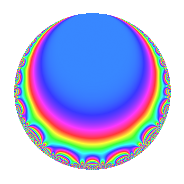Properties

 Label 189.2.i.aLevel 189 Weight 2 Character orbit 189.i Analytic conductor 1.509 Analytic rank 0 Dimension 2 CM No Inner twists 2

Related objects

Newspace parameters

 Level: $$N$$ = $$189 = 3^{3} \cdot 7$$ Weight: $$k$$ = $$2$$ Character orbit: $$[\chi]$$ = 189.i (of order $$6$$ and degree $$2$$)

Newform invariants

 Self dual: No Analytic conductor: $$1.5091725982$$ Analytic rank: $$0$$ Dimension: $$2$$ Coefficient field: $$\Q(\sqrt{-3})$$ Coefficient ring: $$\Z[a_1, \ldots, a_{5}]$$ Coefficient ring index: $$1$$ Sato-Tate group: $\mathrm{SU}(2)[C_{6}]$

$q$-expansion

Coefficients of the $$q$$-expansion are expressed in terms of a primitive root of unity $$\zeta_{6}$$. We also show the integral $$q$$-expansion of the trace form.

 $$f(q)$$ $$=$$ $$q$$ $$+ ( -1 + 2 \zeta_{6} ) q^{2}$$ $$- q^{4}$$ $$+ ( 3 - 3 \zeta_{6} ) q^{5}$$ $$+ ( 1 + 2 \zeta_{6} ) q^{7}$$ $$+ ( -1 + 2 \zeta_{6} ) q^{8}$$ $$+O(q^{10})$$ $$q$$ $$+ ( -1 + 2 \zeta_{6} ) q^{2}$$ $$- q^{4}$$ $$+ ( 3 - 3 \zeta_{6} ) q^{5}$$ $$+ ( 1 + 2 \zeta_{6} ) q^{7}$$ $$+ ( -1 + 2 \zeta_{6} ) q^{8}$$ $$+ ( 3 + 3 \zeta_{6} ) q^{10}$$ $$+ ( -2 + \zeta_{6} ) q^{11}$$ $$+ ( 2 - \zeta_{6} ) q^{13}$$ $$+ ( -5 + 4 \zeta_{6} ) q^{14}$$ $$-5 q^{16}$$ $$+ ( 3 - 3 \zeta_{6} ) q^{17}$$ $$+ ( -6 + 3 \zeta_{6} ) q^{19}$$ $$+ ( -3 + 3 \zeta_{6} ) q^{20}$$ $$-3 \zeta_{6} q^{22}$$ $$+ ( -3 - 3 \zeta_{6} ) q^{23}$$ $$-4 \zeta_{6} q^{25}$$ $$+ 3 \zeta_{6} q^{26}$$ $$+ ( -1 - 2 \zeta_{6} ) q^{28}$$ $$+ ( 3 + 3 \zeta_{6} ) q^{29}$$ $$+ ( 2 - 4 \zeta_{6} ) q^{31}$$ $$+ ( 3 - 6 \zeta_{6} ) q^{32}$$ $$+ ( 3 + 3 \zeta_{6} ) q^{34}$$ $$+ ( 9 - 3 \zeta_{6} ) q^{35}$$ $$-7 \zeta_{6} q^{37}$$ $$-9 \zeta_{6} q^{38}$$ $$+ ( 3 + 3 \zeta_{6} ) q^{40}$$ $$+ 3 \zeta_{6} q^{41}$$ $$+ ( -1 + \zeta_{6} ) q^{43}$$ $$+ ( 2 - \zeta_{6} ) q^{44}$$ $$+ ( 9 - 9 \zeta_{6} ) q^{46}$$ $$+ ( -3 + 8 \zeta_{6} ) q^{49}$$ $$+ ( 8 - 4 \zeta_{6} ) q^{50}$$ $$+ ( -2 + \zeta_{6} ) q^{52}$$ $$+ ( -5 - 5 \zeta_{6} ) q^{53}$$ $$+ ( -3 + 6 \zeta_{6} ) q^{55}$$ $$+ ( -5 + 4 \zeta_{6} ) q^{56}$$ $$+ ( -9 + 9 \zeta_{6} ) q^{58}$$ $$+ ( 8 - 16 \zeta_{6} ) q^{61}$$ $$+ 6 q^{62}$$ $$- q^{64}$$ $$+ ( 3 - 6 \zeta_{6} ) q^{65}$$ $$-4 q^{67}$$ $$+ ( -3 + 3 \zeta_{6} ) q^{68}$$ $$+ ( -3 + 15 \zeta_{6} ) q^{70}$$ $$+ ( 2 - 4 \zeta_{6} ) q^{71}$$ $$+ ( -3 - 3 \zeta_{6} ) q^{73}$$ $$+ ( 14 - 7 \zeta_{6} ) q^{74}$$ $$+ ( 6 - 3 \zeta_{6} ) q^{76}$$ $$+ ( -4 - \zeta_{6} ) q^{77}$$ $$+ 8 q^{79}$$ $$+ ( -15 + 15 \zeta_{6} ) q^{80}$$ $$+ ( -6 + 3 \zeta_{6} ) q^{82}$$ $$+ ( -15 + 15 \zeta_{6} ) q^{83}$$ $$-9 \zeta_{6} q^{85}$$ $$+ ( -1 - \zeta_{6} ) q^{86}$$ $$-3 \zeta_{6} q^{88}$$ $$+ 3 \zeta_{6} q^{89}$$ $$+ ( 4 + \zeta_{6} ) q^{91}$$ $$+ ( 3 + 3 \zeta_{6} ) q^{92}$$ $$+ ( -9 + 18 \zeta_{6} ) q^{95}$$ $$+ ( 1 + \zeta_{6} ) q^{97}$$ $$+ ( -13 + 2 \zeta_{6} ) q^{98}$$ $$+O(q^{100})$$ $$\operatorname{Tr}(f)(q)$$ $$=$$ $$2q$$ $$\mathstrut -\mathstrut 2q^{4}$$ $$\mathstrut +\mathstrut 3q^{5}$$ $$\mathstrut +\mathstrut 4q^{7}$$ $$\mathstrut +\mathstrut O(q^{10})$$ $$2q$$ $$\mathstrut -\mathstrut 2q^{4}$$ $$\mathstrut +\mathstrut 3q^{5}$$ $$\mathstrut +\mathstrut 4q^{7}$$ $$\mathstrut +\mathstrut 9q^{10}$$ $$\mathstrut -\mathstrut 3q^{11}$$ $$\mathstrut +\mathstrut 3q^{13}$$ $$\mathstrut -\mathstrut 6q^{14}$$ $$\mathstrut -\mathstrut 10q^{16}$$ $$\mathstrut +\mathstrut 3q^{17}$$ $$\mathstrut -\mathstrut 9q^{19}$$ $$\mathstrut -\mathstrut 3q^{20}$$ $$\mathstrut -\mathstrut 3q^{22}$$ $$\mathstrut -\mathstrut 9q^{23}$$ $$\mathstrut -\mathstrut 4q^{25}$$ $$\mathstrut +\mathstrut 3q^{26}$$ $$\mathstrut -\mathstrut 4q^{28}$$ $$\mathstrut +\mathstrut 9q^{29}$$ $$\mathstrut +\mathstrut 9q^{34}$$ $$\mathstrut +\mathstrut 15q^{35}$$ $$\mathstrut -\mathstrut 7q^{37}$$ $$\mathstrut -\mathstrut 9q^{38}$$ $$\mathstrut +\mathstrut 9q^{40}$$ $$\mathstrut +\mathstrut 3q^{41}$$ $$\mathstrut -\mathstrut q^{43}$$ $$\mathstrut +\mathstrut 3q^{44}$$ $$\mathstrut +\mathstrut 9q^{46}$$ $$\mathstrut +\mathstrut 2q^{49}$$ $$\mathstrut +\mathstrut 12q^{50}$$ $$\mathstrut -\mathstrut 3q^{52}$$ $$\mathstrut -\mathstrut 15q^{53}$$ $$\mathstrut -\mathstrut 6q^{56}$$ $$\mathstrut -\mathstrut 9q^{58}$$ $$\mathstrut +\mathstrut 12q^{62}$$ $$\mathstrut -\mathstrut 2q^{64}$$ $$\mathstrut -\mathstrut 8q^{67}$$ $$\mathstrut -\mathstrut 3q^{68}$$ $$\mathstrut +\mathstrut 9q^{70}$$ $$\mathstrut -\mathstrut 9q^{73}$$ $$\mathstrut +\mathstrut 21q^{74}$$ $$\mathstrut +\mathstrut 9q^{76}$$ $$\mathstrut -\mathstrut 9q^{77}$$ $$\mathstrut +\mathstrut 16q^{79}$$ $$\mathstrut -\mathstrut 15q^{80}$$ $$\mathstrut -\mathstrut 9q^{82}$$ $$\mathstrut -\mathstrut 15q^{83}$$ $$\mathstrut -\mathstrut 9q^{85}$$ $$\mathstrut -\mathstrut 3q^{86}$$ $$\mathstrut -\mathstrut 3q^{88}$$ $$\mathstrut +\mathstrut 3q^{89}$$ $$\mathstrut +\mathstrut 9q^{91}$$ $$\mathstrut +\mathstrut 9q^{92}$$ $$\mathstrut +\mathstrut 3q^{97}$$ $$\mathstrut -\mathstrut 24q^{98}$$ $$\mathstrut +\mathstrut O(q^{100})$$

Character Values

We give the values of $$\chi$$ on generators for $$\left(\mathbb{Z}/189\mathbb{Z}\right)^\times$$.

 $$n$$ $$29$$ $$136$$ $$\chi(n)$$ $$\zeta_{6}$$ $$\zeta_{6}$$

Embeddings

For each embedding $$\iota_m$$ of the coefficient field, the values $$\iota_m(a_n)$$ are shown below.

For more information on an embedded modular form you can click on its label.

Label $$\iota_m(\nu)$$ $$a_{2}$$ $$a_{3}$$ $$a_{4}$$ $$a_{5}$$ $$a_{6}$$ $$a_{7}$$ $$a_{8}$$ $$a_{9}$$ $$a_{10}$$
143.1
 0.5 + 0.866025i 0.5 − 0.866025i
1.73205i 0 −1.00000 1.50000 2.59808i 0 2.00000 + 1.73205i 1.73205i 0 4.50000 + 2.59808i
152.1 1.73205i 0 −1.00000 1.50000 + 2.59808i 0 2.00000 1.73205i 1.73205i 0 4.50000 2.59808i
 $$n$$: e.g. 2-40 or 990-1000 Significant digits: Format: Complex embeddings Normalized embeddings Satake parameters Satake angles

Inner twists

Char. orbit Parity Mult. Self Twist Proved
1.a Even 1 trivial yes
63.i Even 1 yes

Hecke kernels

This newform can be constructed as the kernel of the linear operator $$T_{2}^{2}$$ $$\mathstrut +\mathstrut 3$$ acting on $$S_{2}^{\mathrm{new}}(189, [\chi])$$.